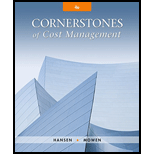# Burnett Company produces two types of gears: Model 12 and Model 15. Market conditions limit the number of each gear that can be sold. For Model 12 no more than 15,000 units can be sold, and for Model 15 no more than 40,000 units. Each gear must be notched by a special machine. Burnett owns eight machines that together provide 60,000 hours of machine time per year. Each unit of Model 12 requires three hours of machine time, and each unit of Model 15 requires 45 minutes or 0.75 hour of machine time. The unit contribution for Model 12 is $60 and for Model 15 is$30. Burnett wants to identify the product mix that will maximize total contribution margin. Required: 1. Formulate Burnett’s problem as a linear programming model. 2. Solve the linear programming model in Requirement 1. 3. Identify which constraints are binding and which are loose. Also, identify the constraints as internal or external.### Cornerstones of Cost Management (C...

4th Edition
Don R. Hansen + 1 other
Publisher: Cengage Learning
ISBN: 9781305970663

#### Solutions

Chapter
Section### Cornerstones of Cost Management (C...

4th Edition
Don R. Hansen + 1 other
Publisher: Cengage Learning
ISBN: 9781305970663
Chapter 20, Problem 24P
Textbook Problem
27 views

## Burnett Company produces two types of gears: Model 12 and Model 15. Market conditions limit the number of each gear that can be sold. For Model 12 no more than 15,000 units can be sold, and for Model 15 no more than 40,000 units. Each gear must be notched by a special machine. Burnett owns eight machines that together provide 60,000 hours of machine time per year. Each unit of Model 12 requires three hours of machine time, and each unit of Model 15 requires 45 minutes or 0.75 hour of machine time. The unit contribution for Model 12 is $60 and for Model 15 is$30. Burnett wants to identify the product mix that will maximize total contribution margin.Required: 1. Formulate Burnett’s problem as a linear programming model. 2. Solve the linear programming model in Requirement 1. 3. Identify which constraints are binding and which are loose. Also, identify the constraints as internal or external.

1.

To determine

Ascertain the formula for a linear programming model of company B.

### Explanation of Solution

Contribution margin: Contribution margin is a measurement of performance where only revenue and variable costs are taken into consideration. Hence, this measurement is useful in the evaluation of the probable outcomes of decisions including pricing decisions and other marketing strategies that affect primarily revenue and variable costs.

Assume that X denotes Model 12 and Y denotes Model 15:

Objective function:

Max Z=$60X+$30Y

Linear programming model:

3X+0.75Y60,000 (1)             &#

2.

To determine

Calculate the linear programming model in Requirement 1.

3.

To determine

Identify the constraints that are binding and that are loose and also state whether the identified constraints are internal or external constraints.

### Still sussing out bartleby?

Check out a sample textbook solution.

See a sample solution

#### The Solution to Your Study Problems

Bartleby provides explanations to thousands of textbook problems written by our experts, many with advanced degrees!

Get Started

Find more solutions based on key concepts
Why are accruals called spontaneous sources of funds, what are their costs, and why dont firms use more of them...

Fundamentals of Financial Management, Concise Edition (with Thomson ONE - Business School Edition, 1 term (6 months) Printed Access Card) (MindTap Course List)

What distinguishes a B2B from a B2C e-business model?

Foundations of Business (MindTap Course List)

What is goal congruence?

Accounting Information Systems